September 9

# Study the Interference Pattern using MATLAB

lang: en_US

## What is interference?

Interference is a fascinating phenomenon that occurs when two waves combine to form a new wave. According to Wikipedia, interference is a phenomenon in which two waves combine by adding their displacement at every single point in space and time to form a resultant wave of greater, lower, or the same amplitude.

Imagine two ripples on a pond coming together - as they meet, they can either reinforce each other and create a larger wave, or cancel each other out and create a calm spot. This is the basic idea behind constructive and destructive interference. These interferences result due to the interaction of waves that are correlated or coherent with each other. Interference effects can be observed with all waves, for example, light, radio, acoustic, surface water, gravity, or matter waves.

Scientists use special tools called interferometers to study interference and make precise measurements. These are essential optical tools used to precisely measure wavelength, distance, refraction index, and optical beams' temporal coherence. One of the most famous interferometers is the Michelson Interferometer, invented by Albert Michelson in 1890.

## The Michelson Interferometer

In the Michelson interferometer, light from a source is split into two beams at a beam splitter (partially reflecting mirror).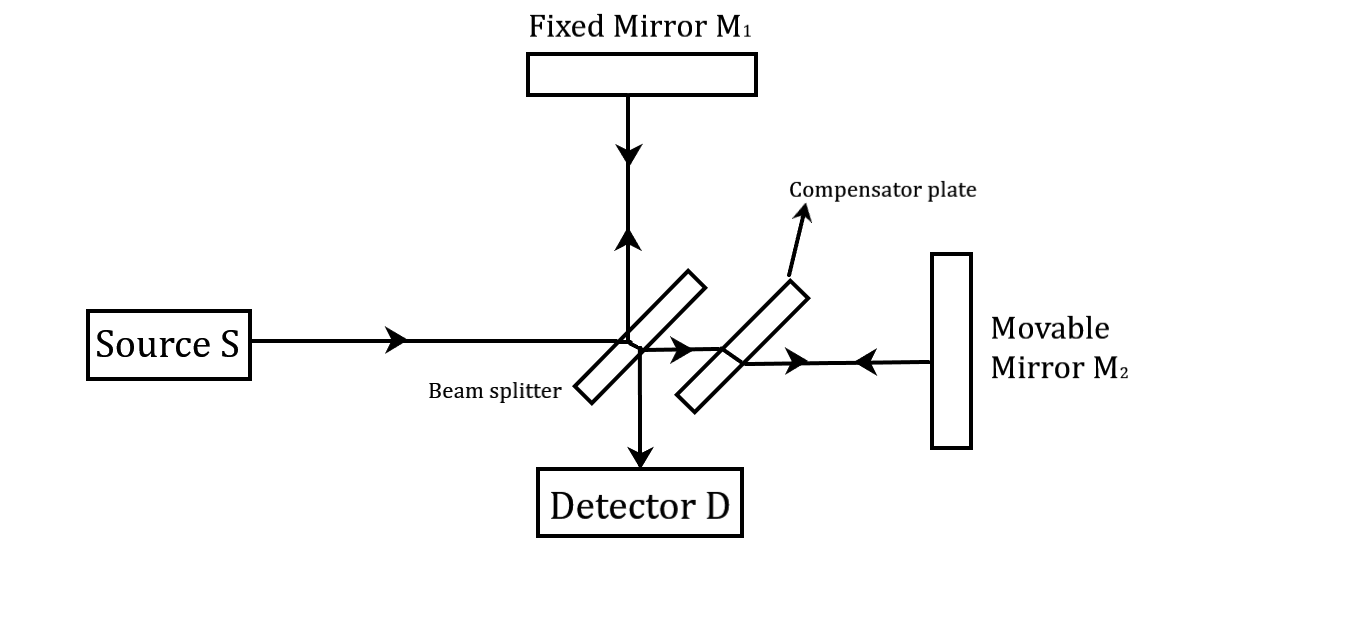Michelson Interferometer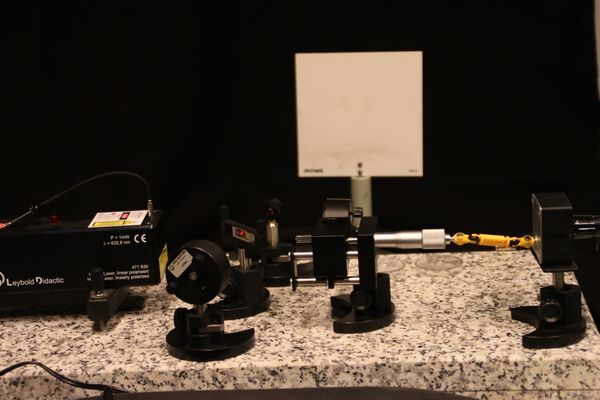Physical apparatus setup of Michelson Interferometer

The light beam travels to the beam splitter, where it is partially reflected and partially transmitted.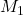and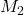are plane mirrors whereis a fixed mirror whileis movable. In normal settings, the mirrorsandare perpendicular to each other while the beam splitter is at an angle of  to the mirror. From the beam splitter, the light goes to a mirrorand is reflected to the beam splitter while the other beam is reflected from the mirrorback to the beam splitter. The two beams recombine and are then detected at the detector.

The two waves will interfere constructively or destructively as per the following conditions of path difference,

Destructive interference gives us a dark spot. It is given by,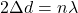Constructive interference gives us a bright spot. It is given by,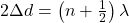## Interference pattern due to monochromatic plane wave

Assume the two mirrorsandis illuminated by an incident plane wave. The mirroris titled at an angle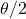for the X axis. Let the Origin of the coordinate system be the centre of mirror. The waves are reflected normally by mirror(wave 1) and at an angle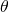to the Z axis by mirror(wave 2).

The electric fields for the two plane waves at the point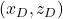on the screen of the detector are,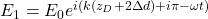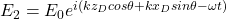The resultant electric field at the detector is given by,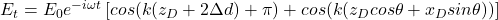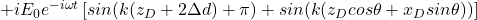The intensity S of the combined beam is given at the detector screen as,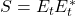which can be solved to get,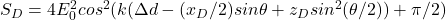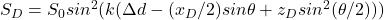hence,## When Mirrors are perpendicular to the beam

If the two mirrors are precisely parallel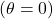, then the intensity on the screen is,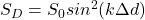and the whole area of the screen will be uniformly illuminated. The image below shows how you can represent this condition.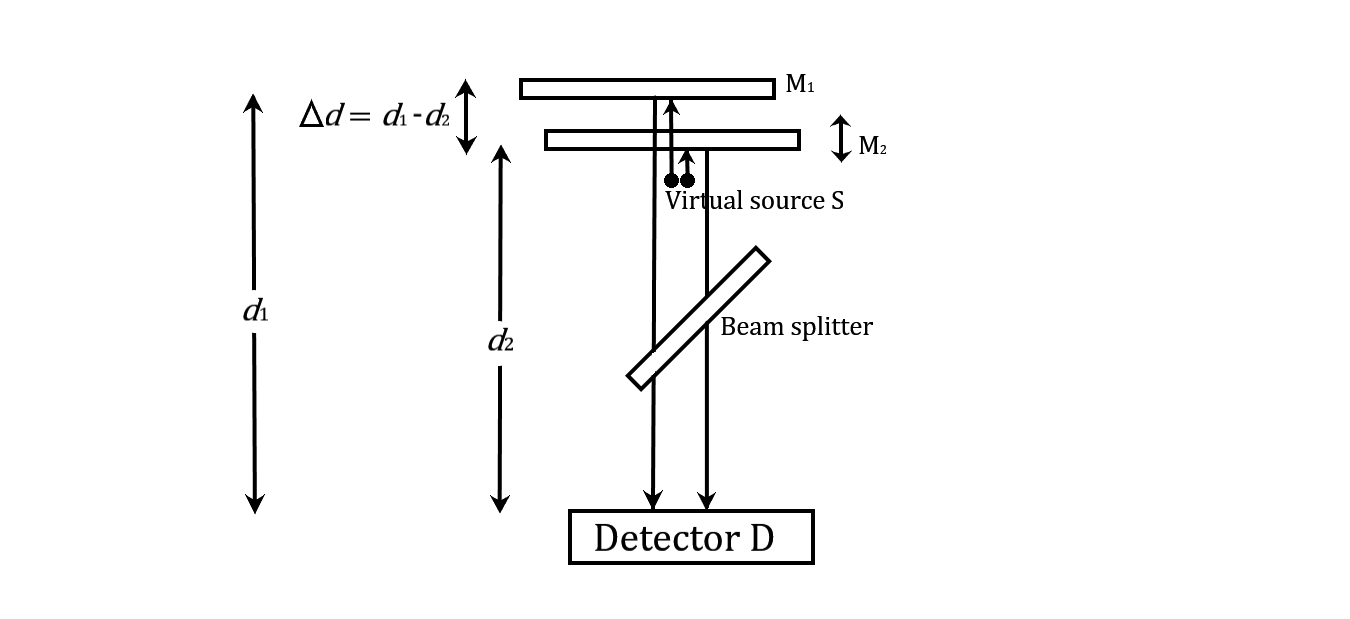Optical equivalent of Michelson Interferometer

The screen will be dark for an integral multiple of a wavelength and bright when there is an odd multiple of a half-wavelength.

## Waves reflected by M2 at some angle

This condition is the commonly studied interference pattern formed by an interferometer. The wavefronts reflected from mirrorare parallel to the screen, while the wavefronts reflected from mirrorare tilted at an angle to the screen. The path difference along the line of intersection is zero and, therefore, is the same for all the wavelengths. When a source of white light is used, we get a central achromatic bright fringe. On either side of the central fringe, few coloured straight fringes are observed.

The fringes are given by following equation,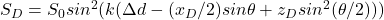Using above equation, the spacing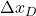between two adjacent bright and dark fringes at positions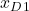and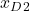is given by,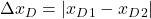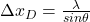When,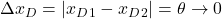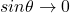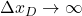The fringes disappear, and the screen becomes uniformly illuminated, as described above.

The fringe separation becomes smaller as the wavelength decreases and the tilt angle becomes larger. When mirroris moved, the fringes move horizontally across the detector screen.

## Modelling the interferometer in MATLAB

Code & Report!

Study the interference pattern formed by two light waves and model a MATLAB version of the Michelson Interferometer; Developed in MATLAB R2018a

Ready to take your MATLAB skills to the next level? Join our free online course of MATLAB Fundamentals and start your journey towards becoming a MATLAB expert! Already familiar with the basics? Let's dive into modelling and simulations!

To model the Michelson interferometer in MATLAB and generate the interference pattern, we first need to write a For example, if the wavelength is 400 nanometres. We will write the code as follows:

function that will convert the input wavelength to its corresponding colour. It is easy and can be achieved using the if – and condition several times.

if (lambda>=380)&&(lambda<440)

thiscolor = [(440-lambda)/(440-380),0,1];

Be sure to change the value of lambda to the nanometre scale. Next, we need to initialise the several variables that will contain information to be used in modelling. It will include data like wavelength, mirror separation distance, angle theta, detector screen dimensions, propagation constants etc.

Now we will use the electric field equations that we described earlier (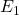and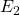). The interference can be performed by simply adding them together as we did earlier with the equation to get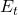. We will also use the detector screen intensity equation obtained by. The final part is to plot the detector screen intensity. It can be achieved using pcolor() and colorMap() function of MATLAB. You might also want to plot a line graph to study the variation in the intensity of the detector screen. We will also print out useful information in the command window like wavelength, mirror separation distance, angle theta and fringe spacing.

Now that we have created the model let's run it and check our result.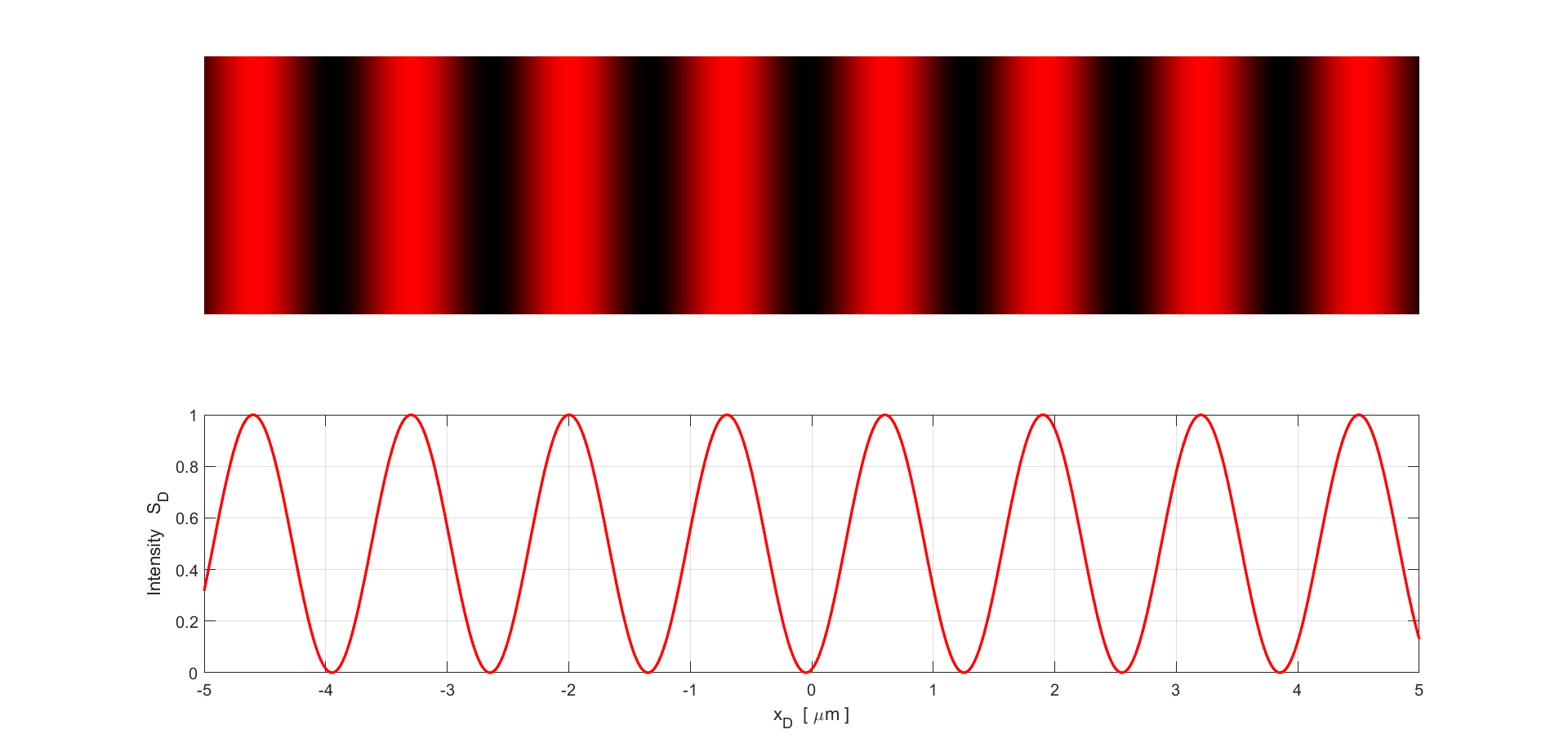Result

Inputs:

wavelength = 650.00 nm

mirror separation distance = 1202.50 nm

X tilt angle = 30.00 deg

Outputs:

fringe spacing = 1.30 um

As you can see, I am using a wavelength of 650 nanometres which corresponds to the wavelength of red light. The mirror separation distance I used is around 1202.5 nanometres, while the angle theta is 30 degrees. The fringe spacing, we got in our plot is approximately 1.3 micrometres. The plot is quite self-explanatory. The intensity is maximum whenever there is constructive interference, and we get bright maxima. In contrast, we get minima whenever there is destructive interference.

## Application of wave interference

Interferometry, the study of wave interference, has had a profound impact on the field of physics and has been widely used in many different areas of measurement. One of the most famous experiments in this field, Young's double slit interferometer, was a major contributor to the acceptance of the wave theory of light and helped to demonstrate the wave-particle duality of light and other quantum particles.

Interferometry is also widely used in acoustics, where it is used to measure the properties of sound waves in gases and liquids. The Michelson interferometer, in particular, is highly accurate and can be used to measure small displacements, such as the growth rate of plants.

Overall, interferometry plays an essential role in our understanding of the physical world and has been widely used in many different areas of measurement. Whether it's studying the properties of light, sound, or even plants, interferometry has the ability to help us make highly accurate measurements that would be otherwise impossible.

Ready to take your MATLAB skills to the next level? Look no further! At MATLAB Helper, we've got you covered. From free community support to expert help and training, we've got all the resources you need to become a pro in no time. If you have any questions or queries, don't hesitate to reach out to us. Simply post a comment below or send us an email at [email protected].

And don't forget to connect with us on LinkedIn, Facebook, and Subscribe to our YouTube Channel! We're always sharing helpful tips and updates, so you can stay up-to-date on everything related to MATLAB. Plus, if you spot any bugs or errors on our website, just let us know and we'll make sure to fix it ASAP.

Ready to get started? Book your expert help with Research Assistance plan today and get personalized assistance tailored to your needs. Or, if you're looking for more comprehensive training, join one of our training modules and get hands-on experience with the latest techniques and technologies. The choice is yours – start learning and growing with MATLAB Helper today!

Education is our future. MATLAB is our feature. Happy MATLABing!

0 shares

Tags

interference, interference due to Michelson interferometer, interference of light, interference of waves, interference pattern, interference physics, michelson interferometer

#### Shubham

I have done my bachelor degree in electronics from University of Delhi. I like to tinker with the hardware to understand how it actually works.

## Modified – Euler’s method in MATLAB

Modified – Euler’s method in MATLAB

## Runge-Kutta method in MATLAB

Runge-Kutta method in MATLAB

#### MATLAB Helper ®

Use Website Chat or WhatsApp at +91-8104622179